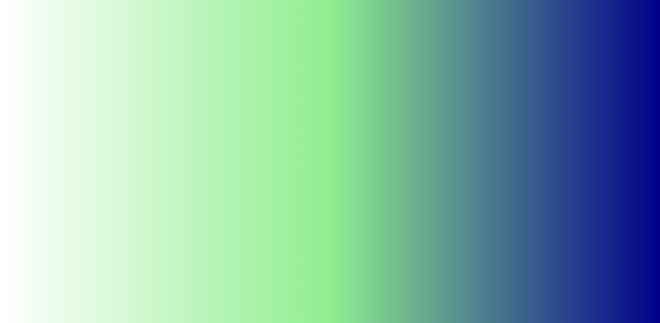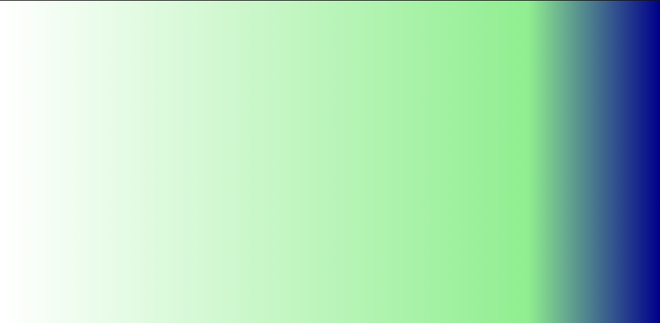GeeksforGeeks App
Open AppBrowser
Continue

# How to create linear gradient background using CSS ?

In CSS, we can use the background-color property to set the background color of an element to a specific color. Sometimes we want to add more styling to the element when setting the background color by using the linear-gradient property. CSS linear-gradient property lets you display smooth transitions between two or more colors.

Syntax:

`background-image: linear-gradient(direction, color1, color2, ...);`

Parameters:

• direction: Specify the direction of the transition. The default value is 180deg (if not specified).
• color1: Specify the first color.
• color2: Specify the second color.

Note: You can specify as many colors as you want.

Example 1: In this example, we are using the above method.

## HTML

 ```<``html``>``<``head``>``    ``<``style``>``        ``/* Remove the default padding and``           ``margin of all HTML tags */``        ``* {``            ``margin: 0;``            ``padding: 0;``        ``}` `        ``/* Implementation of linear-gradient property */``        ``#lin_grad {` `            ``/* Set the height of the div to``               ``the entire screen */``            ``height: 100vh;` `            ``/* linear-gradient syntax */``            ``background-image: linear-gradient(lightblue, darkblue);``        ``}``    ````` `<``body``>``    ``<``div` `id``=``"lin_grad"``>`````

Output:Example 2: This demonstrates the setting of direction. In the above HTML code, just change the “background-image” property value in the CSS part of the head section as given below.

Syntax:

`background-image: linear-gradient(to right, lightblue, darkblue)`

Example: Here we are following above explained approach.

## HTML

 ```<``html``>``<``head``>``    ``<``style``>``        ``/* Remove the default padding and``        ``margin of all HTML tags */``        ``* {``            ``margin: 0;``            ``padding: 0;``        ``}` `        ``/* Implementation of linear-gradient property */``        ``#lin_grad {` `            ``/* Set the height of the div to``            ``the entire screen */``            ``height: 100vh;` `            ``/* linear-gradient syntax */``            ``background-image: linear-gradient(to right, lightblue, darkblue)``        ``}``    ````` `<``body``>``    ``<``div` `id``=``"lin_grad"``>`````

Output:Example 3: This demonstrates the setting of direction in degree. In the above HTML first code, just change the “background-image” property value in the CSS part of the head section as given below.

Syntax:

`background-image: linear-gradient(135deg, white, lightgreen, darkblue);`

Example: Here we are using the above syntax.

## HTML

 ```<``html``>``<``head``>``    ``<``style``>``        ``/* Remove the default padding and``        ``margin of all HTML tags */``        ``* {``            ``margin: 0;``            ``padding: 0;``        ``}` `        ``/* Implementation of linear-gradient property */``        ``#lin_grad {` `            ``/* Set the height of the div to``            ``the entire screen */``            ``height: 100vh;` `            ``/* linear-gradient syntax */``            ``background-image: linear-gradient(135deg, white, lightgreen, darkblue);``        ``}``    ````` `<``body``>``    ``<``div` `id``=``"lin_grad"``>`````

Output:Example 4: This demonstrates the setting of the position for each color. If you don’t specify the location of a color, it is placed halfway between its preceding color and the color that follows it. The two gradients given below are equivalent. In the above HTML first code, just change the “background-image” in the CSS part of the head section as given below.

Syntax:

```background-image: linear-gradient(90deg, white, lightgreen, darkblue)
lightgreen 50%, darkblue 100%)```

## HTML

 ```<``html``>``<``head``>``    ``<``style``>``        ``/* Remove the default padding and``        ``margin of all HTML tags */``        ``* {``            ``margin: 0;``            ``padding: 0;``        ``}` `        ``/* Implementation of linear-gradient property */``        ``#lin_grad {` `            ``/* Set the height of the div to``            ``the entire screen */``            ``height: 100vh;` `            ``/* linear-gradient syntax */``            ``background-image:``                  ``linear-gradient(90deg, white, lightgreen, darkblue)``                  ``background-image: linear-gradient(90deg, white 0%,``                    ``lightgreen 50%, darkblue 100%)``        ``}``    ````` `<``body``>``    ``<``div` `id``=``"lin_grad"``>`````

Output:Let us change the % value of the light green color and see what happens.

Syntax:

```background-image: linear-gradient(90deg, white 0%,
lightgreen 80%, darkblue 100%)```

Example:

## HTML

 ```<``html``>``<``head``>``    ``<``style``>``        ``/* Remove the default padding and``        ``margin of all HTML tags */``        ``* {``            ``margin: 0;``            ``padding: 0;``        ``}` `        ``/* Implementation of linear-gradient property */``        ``#lin_grad {` `            ``/* Set the height of the div to``            ``the entire screen */``            ``height: 100vh;` `            ``/* linear-gradient syntax */``            ``background-image: background-image: linear-gradient(90deg, white 0%,``                    ``lightgreen 80%, darkblue 100%)``        ``}``    ````` `<``body``>``    ``<``div` `id``=``"lin_grad"``>`````

Output:My Personal Notes arrow_drop_up
Related Tutorials